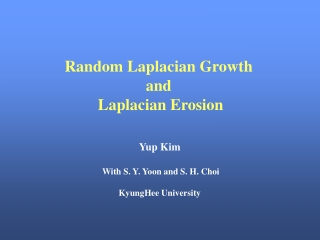Download PresentationRandom Laplacian Growth and Laplacian Erosion

# Random Laplacian Growth and Laplacian Erosion

Télécharger la présentation## Random Laplacian Growth and Laplacian Erosion

- - - - - - - - - - - - - - - - - - - - - - - - - - - E N D - - - - - - - - - - - - - - - - - - - - - - - - - - -
##### Presentation Transcript

1. Random Laplacian Growth and Laplacian Erosion Yup Kim With S. Y. Yoon and S. H. Choi KyungHee University

2. 1. Why Laplacian? • a) Normal Random Walk • b) Ballistic Motion • c) Biased Random Walk ( Diffusion Equation ) (Completely Drifted) (Drifted-Diffusion Equation) Click

3. 2. Three Paradigms for Random, • Irreversible and Nonequilibrium Growth • a) Ballistic Deposition • 1) No Anomalous Internal Struture • 2) Nontrivial Surface Structure • (Dynamical Scaling Law : =1/2, =1/3, z=3/2 (1d)) • KPZ equation Click

4. b) Diffusion-Limited Deposition 1) finitely-ramified fractal structure 2) Click

5. c) Eden Growth (Dynamical Scaling Law : =1/2, =1/3, z=3/2 (1d)) KPZ equation Click

6. Family model Ballistic Erosion • 3. Corresponding Erosion Models • to the Three Paradigms • a) Ballistic Erosion • (Dynamical Scaling Law : =1/2, =1/4, z=2 (1d)) • EW Equation : Linear Equation Click

7. b) Diffusion-Limited Erosion Model • (Dynamical Scaling Law : z=1) • c) Anti-Eden Model •  Critically the same as original Eden Model Click Eden Model Anti-Eden Model

8. Abandoned particle Killing line yk Starting line (1-Pb)/3 (1-Pb)/3 (1-Pb)/3 ys Pb Hopping Probability ymax 4. Biased Diffusion Limited Erosion a) Model L

9. b) Corresponding Continuum Equation and Scaling Property <(x, t)>=0 (Dynamical Scaling Law : z=1)

10. c) Simulation Results Pb = 0.25 (DLD) Dynamic Scaling Law : z = 1

11. Pb = 0.26  = 0.11 Dynamic Scaling Law : z = 1.6

12. Pb = 0.73  = 0.51 Dynamic Scaling Law :  = 0.51 (1/2),  = 0.24 (1/4), z = 2.1 (2)

13.  Scaling Property Summary Growth exponent  Rougheness exponent  Dynamic exponent z

14. Pb = 0.2 Dynamic Scaling Law : z = 1

15. d) Summary Biased diffusion Limited Erosions have three regimes.  Regime I : Pb 0.16 : smooth phase (no roughening)  Regime II : 0.17  Pb  0.25 : z=1  Regime III : Pb  0.25 : z2 (EW universality class) The crossover from regime II to regime III is very sudden.

16. DF 0 2 0.5 1.89 ± 0.01 1 1.75 ±0.02 2 ~1.6a 5. Dielectric Breakdown Model and Anti-dielectric Breakdown Model a) Dielectric Breakdown Model Laplacian Equation (Slow process) Boundary Condition :  (x, y, t) = 0and  (x, yb, t) = 1  Relaxation Method Growth Probability

17.  (x, yb, t) = 1 yb (x,h)  (x, y, t) = 0 b) Anti-dielectric Breakdown Model Erosion Probability

18. c) Simulation Results = 1  비교 Diffusion Limited Annihilation (Pb= 0.25)

19. < 1   = 0 (Anti-Eden Model)  = 0.33  = 0.49 Dynamic Scaling Law :  = 1/2,  = 1/3, z = 3/2(1.49)

20.   = 0.01  = 0.48  = 0.33 Dynamic Scaling Law :  = 0.48 (1/2),  = 0.33 (1/3), z = 1.45 (3/2)

21.   = 0.1  = 0.30  = 0.18 Crossover Regime : z = 1.67

22.  >1 ( = 2)   = 0.5 Dynamic Scaling Law : z = 1

23. d) Summary   0 anti-Eden model (KPZ equation) 0.1<  < 0.5(?) Crossover Regime(?) EW or KPZ universality class(?) 0.5   2 Linear growth equation(Diffusion Limited Erosion) Dynamic exponent, z=1  >2(?)   : smooth phase (No roughening)

24. Erosion Model with Drifted-Laplacian Equation * ? + Boundary Condition D=0, Ballistic Motion ? Poisson Equation ? Source term ? 6. Final Discussion